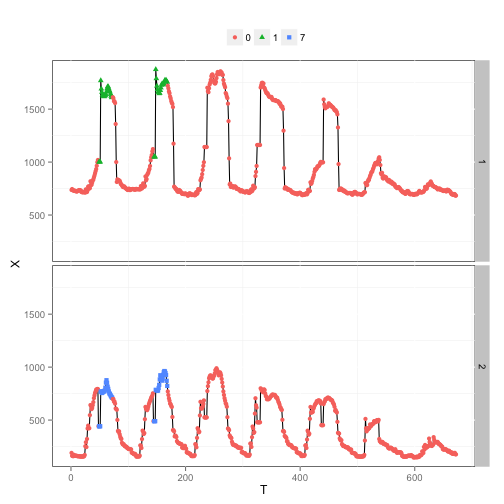title: “Mining Univariate and Multivariate Motifs in Time-Series Data” author: “Cheng Fan” date: “June 25, 2015”

## output: html_document

#Section 1: Overview

This short tutorial guides the users to perform univariate and multivariate motif discovery in time-series data using the “TSMining” package in R. The univariate motif discovery method implemented was proposed by Chiu, Keogh and Lonardi in 2003. The multivariate motif discovery method implemented was proposed by Vahdatpour, Amini and Sarrafzadeh in 2009. It should be noted that the symbolic approximation aggregate (SAX) method is also included in this package for data pre-processing. The SAX method transforms the numeric time series into symbols. The motif discovery methods are based on SAX symbols, rather than the original time-series data. Another two useful functions included in this package are “Func.visual.SingleMotif” and “Func.visual.MultiMotif”. These two functions are used for the ease of visualizing the motifs discovered.

The example data to be used in this tutorial is called “BuildOperation” and also included in the package. It is a one-week data set (the data are collected at an interval of 15-minute) containing the power consumption data of two building subsystems, i.e., the water-cooled chillers (WCC) and air-hanling unit (AHU). These two sub-systems are the key component in building air-conditioning system. The aim is to discover both the univariate and multivariate motifs in the power consumptions of these two sub-systems. The summary of the data are shown as below

The summary of the data are shown as below:

##      Month            Day          Hour           Minute
##  Min.   :2.000   Min.   : 1   Min.   : 0.00   Min.   : 0.00
##  1st Qu.:2.000   1st Qu.: 2   1st Qu.: 5.75   1st Qu.:11.25
##  Median :2.000   Median :25   Median :11.50   Median :22.50
##  Mean   :2.286   Mean   :19   Mean   :11.50   Mean   :22.50
##  3rd Qu.:3.000   3rd Qu.:27   3rd Qu.:17.25   3rd Qu.:33.75
##  Max.   :3.000   Max.   :28   Max.   :23.00   Max.   :45.00
##       WCC              AHU
##  Min.   : 681.8   Min.   :145.9
##  1st Qu.: 723.4   1st Qu.:204.0
##  Median : 770.1   Median :300.3
##  Mean   : 993.7   Mean   :416.3
##  3rd Qu.:1161.4   3rd Qu.:649.5
##  Max.   :1875.0   Max.   :989.7


#Section 2: Univariate motif discovery

The data were collected at an interval of 15-minute and therefore, each daily records have 96 observations. The first step is to determine the desired motif length. Assuming that we are trying to find motifs with a length of 6-hour, the sliding window size should be 24. In this example, the subsequences are created without overlaps. In other words, the time-series are equally divided into 6-hour segments. If one wants to create subsequences with overlaps, it can be controlled by \emph{overlap} in function \emph{Func.motif}. Here, it is desired to find motifs based on both the shape and absolute values of power consumption data. Hence, only global normalization is used, but not local normalization. Supposing that we want each SAX symbol to represent the power consumption in 1-hour, each subsequence will be represented by 6 SAX symbols (i.e., the word size is 6). The alphabet size is usually set no less than 3 to better preserve the information embedded in the original numeric data. It should be noted that the larger the alphabet size and the larger the word size, the better the resolution can be achieved using SAX transformation. However, the numerotity reduction effect will be weakended. This may negatively affect the computational efficiency.

Another important parameter is the mask size used when performing random projection. It should be smaller than the word size. The mask size should be set according to the tolerance in regarding two subsequences as identical. For instance, if the word size is set as 6 and mask size is 4, two subsequences identified as identical should differs at one position at most. The result reliability and quality in motif discovery also depends on the process of motif candidate identification, motif member identification and so on. These can be controlled by the parameters in the function \emph{max.dist.ratio, count.ratio.1, count.ratio.2}. The motif discovery process is rather subjective and users may need to try out different parameter settings before getting the best results. Interested users may find more detailed information in [Chiu, Keogh and Lonardi, 2003].

Here, the univariate motif discovery is performed for both WCC and AHU power consumption data. The code is shown as follows:

res.wcc <- Func.motif(ts = BuildOperation$WCC, global.norm = T, local.norm = F, window.size = 24, overlap = 0, w = 6, a = 5, mask.size = 5, max.dist.ratio = 1.2, count.ratio.1 = 1.1, count.ratio.2 = 1.1) res.ahu <- Func.motif(ts = BuildOperation$AHU, global.norm = T, local.norm = F, window.size = 24, overlap = 0, w = 6, a = 5, mask.size = 5, max.dist.ratio = 1.2, count.ratio.1 = 1.1, count.ratio.2 = 1.1)


The visualization of the motifs discovered can be easily achieved using the \emph{ggplot2} package and the function \emph{Func.visual.SingleMotif} included in this package. More specifically, the \emph{Func.visual.SingleMotif} function prepares a list of two elements.The first element is a data frame and it can be used to plot the general information of motifs discovered in the whole time series. The second element is a list and its length is equal to the number of motifs discovered in the univariate time series. The element of the list is a data frame containing the original subsequences for each motif. To illustrate, the following code chunk uses the first element to present the general information of motifs discovered.

library(ggplot2)
#Visualization
data.wcc <- Func.visual.SingleMotif(single.ts = BuildOperation$WCC, window.size = 24, motif.indices = res.wcc$Indices)
data.ahu <- Func.visual.SingleMotif(single.ts = BuildOperation$AHU, window.size = 24, motif.indices = res.ahu$Indices)

#Determine the total number of motifs discovered in the time series of WCC
n <- length(unique(data.wcc$data.1$Y))
#Make the plot
ggplot(data = data.wcc$data.1) + geom_line(aes(x = 1:dim(data.wcc$data.1), y = X)) +
geom_point(aes(x = 1:dim(data.wcc$data.1), y = X, color=Y, shape=Y))+ scale_shape_manual(values = seq(from = 1, to = n)) + guides(shape=guide_legend(nrow = 2)) + xlab("Time (15-min)") + ylab("WCC Power Consumption (kW)") + theme(panel.background=element_rect(fill = "white", colour = "black"), legend.position="top", legend.title=element_blank())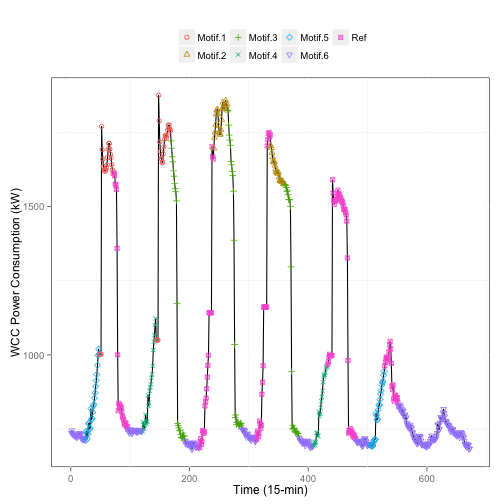#Determine the total number of motifs discovered in the time series of AHU n <- length(unique(data.ahu$data.1$Y)) #Make the plot ggplot(data = data.ahu$data.1) +
geom_line(aes(x = 1:dim(data.ahu$data.1), y = X)) + geom_point(aes(x = 1:dim(data.ahu$data.1), y = X, color=Y, shape=Y))+
scale_shape_manual(values = seq(from = 1, to = n)) +
guides(shape=guide_legend(nrow = 2)) +
xlab("Time (15-min)") + ylab("AHU Power Consumption (kW)") +
theme(panel.background=element_rect(fill = "white", colour = "black"),
legend.position="top",
legend.title=element_blank())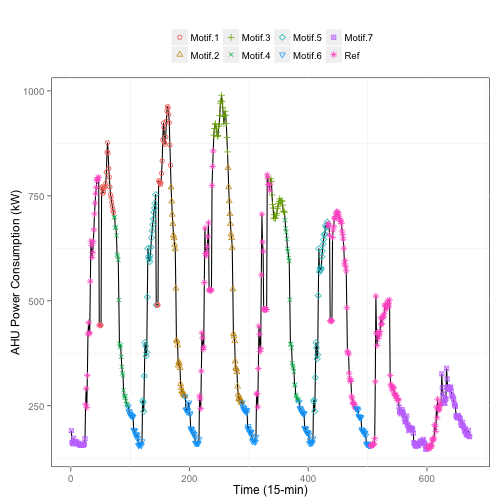The following code chunk uses the second element to visualize the subsequences in each motif discovered in WCC.

for(i in 1:length(data.wcc$data.2)) { data.temp <- data.wcc$data.2[[i]]
print(ggplot(data = data.temp) +
geom_line(aes(x = Time, y = Value, color=Instance, linetype=Instance)) +
xlab("Time (15-min)") + ylab("WCC Power Consumption (kW)") + ggtitle(paste0("WCC Motif ",i)) +
scale_y_continuous(limits=c(0,max(data.temp$Value))) + theme(panel.background=element_rect(fill = "white", colour = "black"), legend.position="none", legend.title=element_blank())) }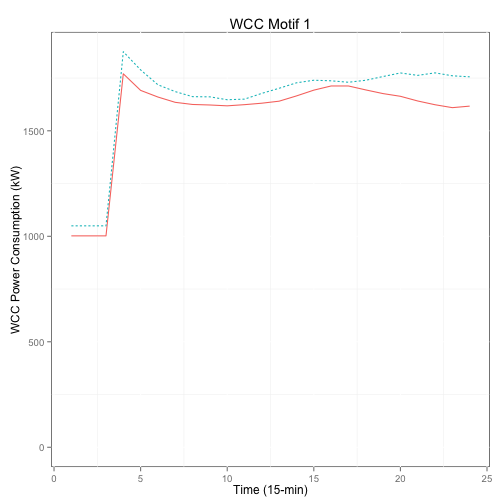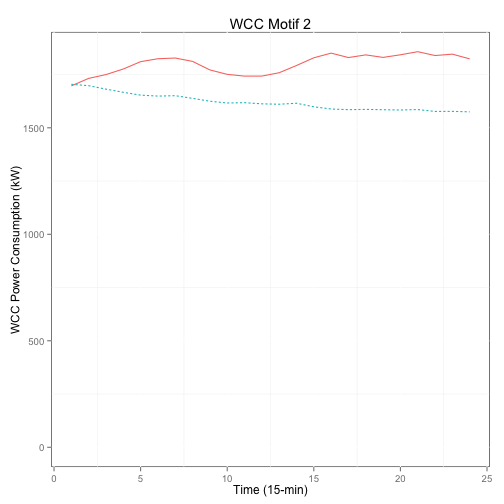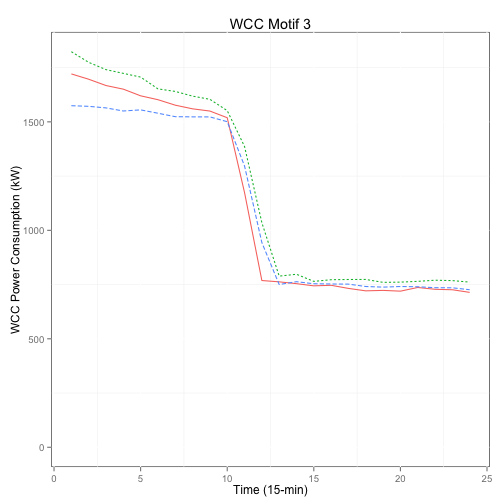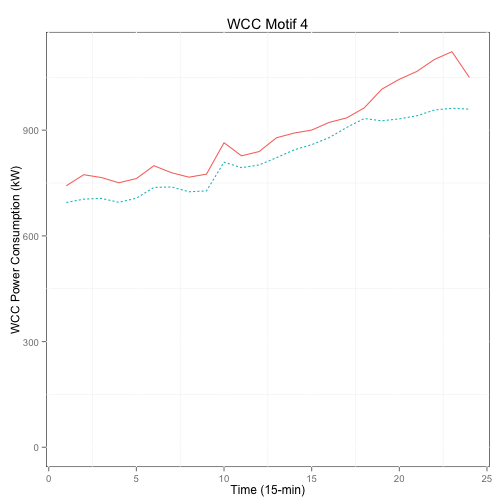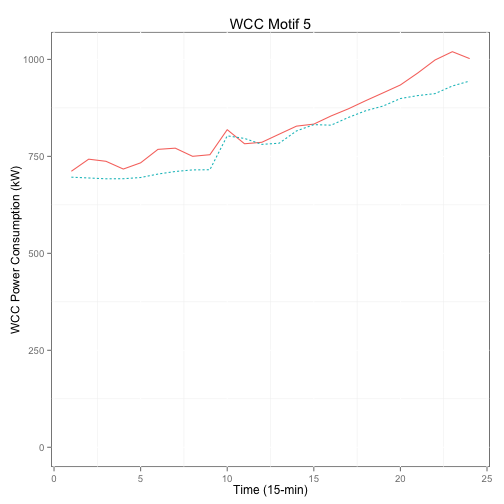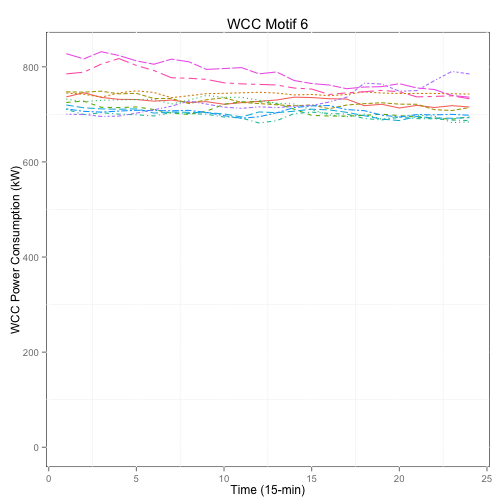The following code chunk uses the second element to visualize the subsequences in each motif discovered in AHU. for(i in 1:length(data.ahu$data.2)) {
data.temp <- data.ahu$data.2[[i]] print(ggplot(data = data.temp) + geom_line(aes(x = Time, y = Value, color=Instance, linetype=Instance)) + xlab("Time (15-min)") + ylab("AHU Power Consumption (kW)") + ggtitle(paste0("AHU Motif ",i)) + scale_y_continuous(limits=c(0,max(data.temp$Value))) +
theme(panel.background=element_rect(fill = "white", colour = "black"),
legend.position="none",
legend.title=element_blank()))
}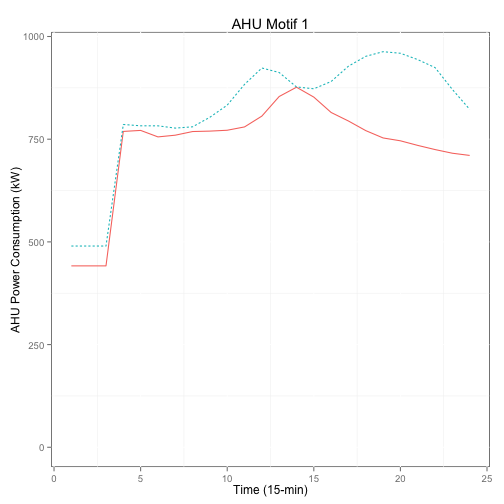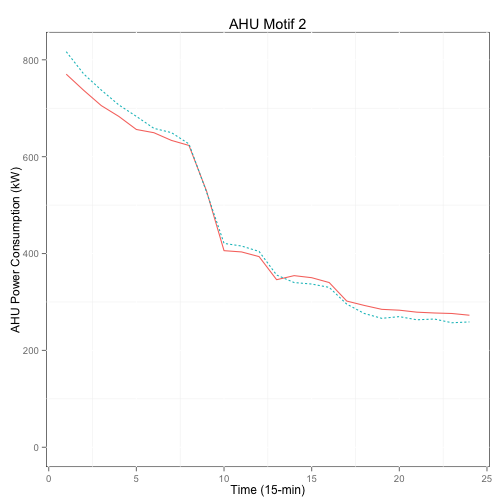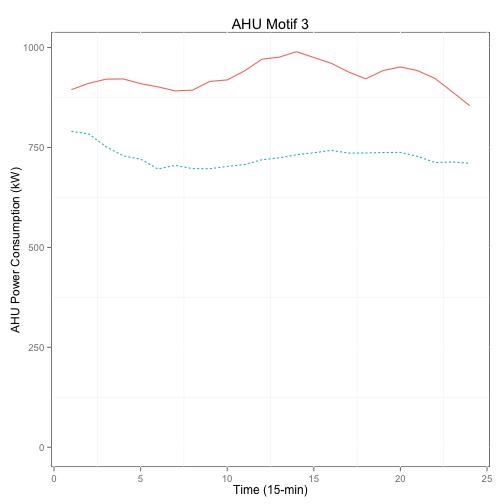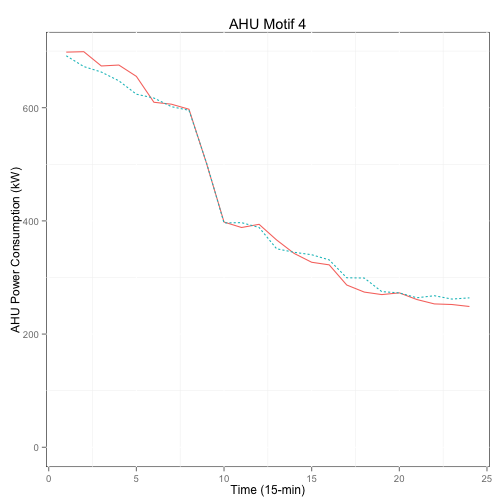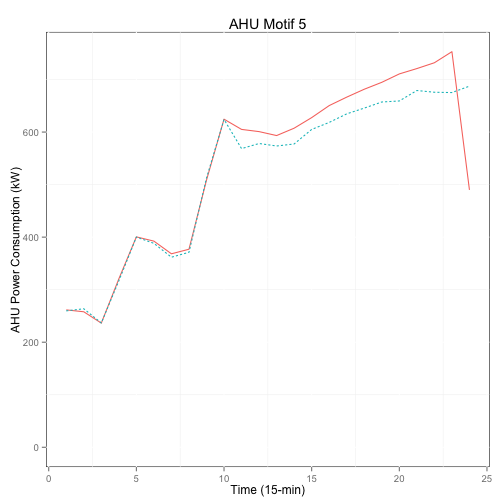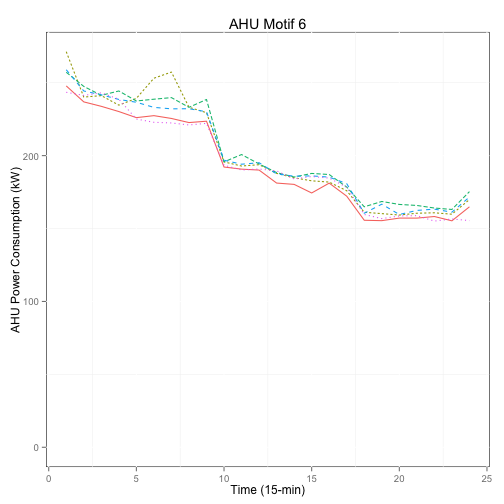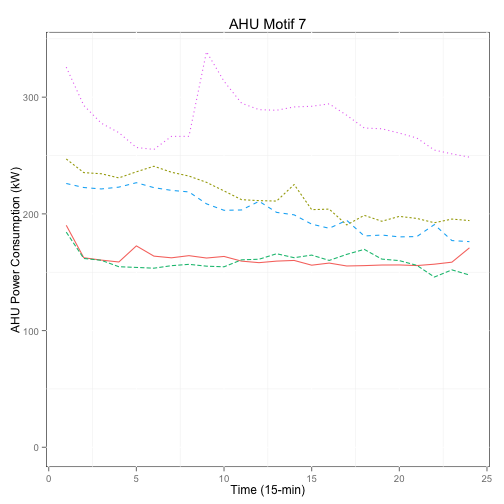#Section 3: Multivariate motif discovery

In this section, the univariate motifs discovered in Section 2 are used to identify multivariate motifs. The function \emph{Func.motif.multivariate} is used to perform this task. The method was proposed by Vahdatpour, Amini and Sarrafzadeh in 2009. The method can successive identify both synchronous and non-synchronous multivariate motifs. Interested users may read their paper for detailed information.

Basically, the function \emph{Func.motif.multivariate} relies on the results of univariate motifs. The first argument of the function takes a list, which has a length of the data dimension. Each element in the list is again a list, which contains the starting position of the subsequences of each motif (this can be extracted from the result returned by function \emph{Func.motif}). The second argument is a vector specifying the window size used to create subsequences in each data dimension. The third argument specifies the minimum correlation threhold before two univariate motifs are considered as a multivariate motif. The following code chunk performs the multivariate motif discovery using the result from Section 2.

res.multi <- Func.motif.multivariate(motif.list = list(res.wcc$Indices, res.ahu$Indices), window.sizes = c(24,24), alpha = .7)

## ===========================================================================


The result can be visualized with the help of function \emph{Func.visual.MultiMotif}. When using this function, a parameter called \emph{index} should be defined. It specifies the No. of multivariate motifs to be considered. The object returned by \emph{Func.visual.MultiMotif} is a data frame containing the information of each multivariate motif. The results can be best presented using the \emph{ggplot2} package. The following graph presents the third multivariate motif. It shows that univariate motif 2 and 9 form a multivariate motif. Univariate motif 2 is from the time series of WCC power consumption (i.e., WCC motif 2) and univariate motif 9 is from the time series of AHU power consumption (i.e., AHU motif 3).

#Focus on the third multivariate motif
data.multi <- Func.visual.MultiMotif(data = BuildOperation[,c("WCC","AHU")], multi.motifs = res.multi, index = 3)

## Joining by: Index.adj

ggplot(data = data.multi, aes(x = T, y = X)) + geom_line() + geom_point(aes(col=Lab, shape=Lab)) + facet_grid(Facet~.) +
theme(panel.background=element_rect(fill = "white", colour = "black"),
legend.title=element_blank(),
legend.position="top")